﻿ 大功率脉冲变压器短路阻抗设计及试验研究 Design and Test Research on Short-Circuit Impedance for High Power Pulse Transformer

Nuclear Science and Technology
Vol. 07  No. 01 ( 2019 ), Article ID: 28288 , 12 pages
10.12677/NST.2019.71003

Design and Test Research on Short-Circuit Impedance for High Power Pulse Transformer

Zhigang Yang1, Yiyun Huang2

1No. 38 Research Institute of CETC, Hefei Anhui

2University of Science and Technology of China, Hefei AnhuiReceived: Dec. 6th, 2018; accepted: Dec. 21st, 2018; published: Dec. 28th, 2018ABSTRACT

In this paper, the short-circuit impedance of HV-MV, HV-LV and MV-LV is analyzed and designed in detail for ITER-PPEN Transformer. The correctness of the design is verified by measuring test on the short-circuit impedance.

Keywords:ITER, PPEN, Transformer, Short-Circuit Impedance

1中国电子科技集团公司第三十八研究所，安徽 合肥

2中国科学技术大学，安徽 合肥Copyright © 2019 by authors and Hans Publishers Inc.

This work is licensed under the Creative Commons Attribution International License (CC BY).

http://creativecommons.org/licenses/by/4.0/1. 引言

ITER (thermo-nuclear experimental reactor)是为解决人类面临的能源短缺、环境污染等问题，由欧、美、日、俄、中、韩、印七方共同启动和参与的一个超大型国际科技合作项目，旨在验证磁约束聚变能科学与工程技术的可行性，是迄今为止我国参加的最大国际合作项目    。ITER所有的部件将由7个参与方分别研制和提供，并按规定时间节点提交安装。经过分解，ITER装置的部件被拆分成22个采购包，共97个子包。我国承担了12个子包(分属6个采购包)的制造任务，预计研制费和加工费达40亿元人民币。PPEN (pulsed power electrical network)采购包是我国承担的任务之一。PPEN主要为ITER装置的磁体电源、微波电源和中性束电源等各类脉冲运行子系统提供脉冲功率，其电能采用法国电网400 kV双进线经3台相同的三相三绕组变压器变电66 kV和22 kV的方式配送，负载分布在66 kV母线和22 kV母线侧，ITER PPEN技术协议书中的主变压器的主要参数如表1所示。Table 1. Main technical parameters of PPEN transformer

2. 短路阻抗的原理

${L}_{\sigma }=\frac{{\phi }_{\sigma }}{I}=\frac{W{\varphi }_{\sigma }}{I}$ (1)

${X}_{\sigma }=\omega {L}_{\sigma }$ (2)

3. 短路阻抗的计算方法( ${R}_{1}$ 为低压绕组内半径； ${R}_{2}$ 为高压绕组外半径； ${r}_{1},{r}_{2}$ 为低压、高压绕组的平均半径； ${r}_{12}$ 为高低压绕组漏磁空道的平均半径； ${a}_{1},{a}_{2}$ 为低压、高压绕组的辐向厚度； ${a}_{12}$ 为高低压绕组漏磁空道的厚度；H为绕组的轴向平均高度)

Figure 1. Magnetic flux distribution schematic diagram for transformer H-L running

$\text{d}{\phi }_{1}=\text{d}{\varphi }_{1}\cdot {w}_{1}\frac{x}{{a}_{1}}=2\text{π}{r}_{1}{B}_{x}\text{d}x\cdot {w}_{1}\frac{x}{{a}_{1}}$ (3)

${\phi }_{1}={\int }_{0}^{{a}_{1}}\text{d}{\phi }_{1}={\int }_{0}^{{a}_{1}}2\text{π}{r}_{1}{B}_{x}\cdot {w}_{1}\frac{x}{{a}_{1}}\text{d}x=\frac{2\text{π}{\mu }_{0}{I}_{1}{W}_{1}^{2}\rho {a}_{1}{r}_{1}}{3H}$ (4)

${\phi }_{2}=\frac{2\text{π}{\mu }_{0}{I}_{2}{W}_{2}^{2}\rho {a}_{2}{r}_{2}}{3H}$ (5)

${\phi }_{12}=\frac{2\text{π}{\mu }_{0}{I}_{1}{W}_{1}^{2}\rho {a}_{12}{r}_{12}}{H}$ (6)

$\phi ={\phi }_{1}+{\phi }_{2}+{\phi }_{12}=\frac{2\text{π}{\mu }_{0}{I}_{1}{W}_{1}^{2}\rho }{H}\left(\frac{{a}_{1}{r}_{1}}{3}+\frac{{a}_{2}{r}_{2}}{3}+{a}_{12}{r}_{12}\right)$ (7)

${x}_{k}=2\text{π}f\frac{2\text{π}{\mu }_{0}{I}_{1}{W}_{1}^{2}\rho \sum D}{H{I}_{1}}=\frac{4{\text{π}}^{2}f{\mu }_{0}{W}_{1}^{2}\rho \sum D}{H}$ (8)

${U}_{kx}=\frac{{u}_{kx}}{{U}_{N}}%=\frac{4{\text{π}}^{2}f{\mu }_{0}{I}_{1}{W}_{1}^{2}\rho \sum D}{H{U}_{N}}%=\frac{4{\text{π}}^{2}f{\mu }_{0}{I}_{1}{W}_{1}\rho \sum D}{H{e}_{t}}%$ (9)

${U}_{k}=K{U}_{kx}=\frac{24.8{I}_{1}{W}_{1}\rho \sum D}{H{e}_{t}×{10}^{4}}%$ (10)

4. PPEN主变压器短路阻抗计算分析

PPEN主变压器的结构由内到外依次是铁芯、低压绕组、中压绕组、高压绕组、分接绕组、油箱。下面按照3节中推导的短路阻抗的工程计算方法，依次求取额定分接高–中、最小分接高–中、最大分接高–中、额定分接高–低、最小分接高–低、最大分接高–低、中–低的短路阻抗。

4.1. 额定分接高–中短路阻抗计算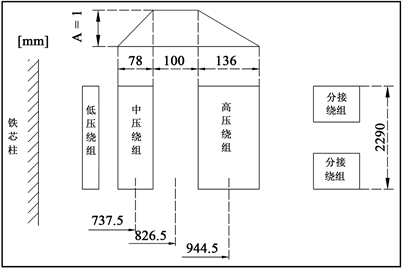Figure 2. Magnetic flux distribution schematic diagram for transformer H-M running in rated tapTable 2. Calculation of the area of magnetic flux leakage for transformer H-M running in rated tap

${{U}^{\prime }}_{kx}=\frac{24.8×0.98×433.03×636×0.956×1446.42}{229×362.9×{10}^{4}}%=11.14%$ (11)

4.2. 最小分接高–中短路阻抗计算

$F={W}_{2}×{I}_{2}×\frac{{U}_{1N}}{{U}_{2N}}=105×\frac{250000}{\sqrt{3}×66}×\frac{300000}{250000}=275553.5$ (12)

${A}_{2}={W}_{F}×{I}_{F}/F=64×481.74/275553.5=0.11$ (13)

${A}_{1}={W}_{1}×{I}_{F}/F=636×481.74/275553.5=1.11$ (14)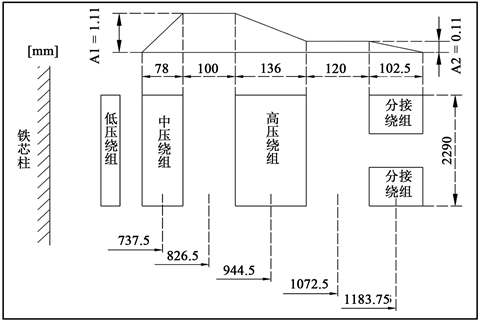Figure 3. Magnetic flux distribution schematic diagram for transformer H-M running in min. tapTable 3. Calculation of the area of magnetic flux leakage for transformer H-M running in min. tap

${{U}^{\prime }}_{kx}=\frac{24.8×0.98×433.03×636×0.925×1565.19}{229×362.9×{10}^{4}}%=11.66%$ (15)

4.3. 最大分接高–中短路阻抗计算

${A}_{2}={W}_{F}×{I}_{F}/F=64×393.65/275553.5=0.09$ (16)

${A}_{1}={W}_{1}×{I}_{F}/F=636×393.65/275553.5=0.91$ (17)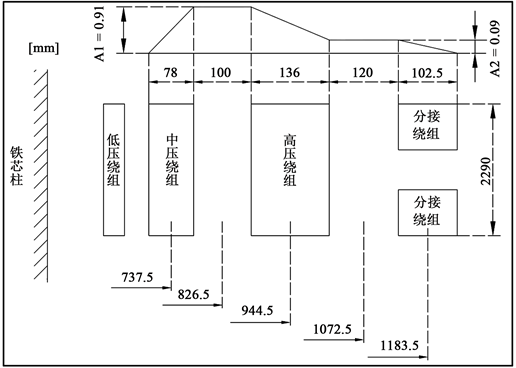Figure 4. Magnetic flux distribution schematic diagram for transformer H-M running in max. tapTable 4. Calculation of the area of magnetic flux leakage for transformer H-M running in max. tap

${{U}^{\prime }}_{kx}=\frac{24.8×0.98×433.03×636×0.925×1387.17}{229×362.9×{10}^{4}}%=10.34%$ (18)

4.4. 额定分接高–低短路阻抗计算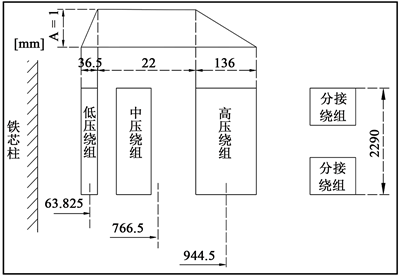Figure 5. Magnetic flux distribution schematic diagram for transformer H-L running in rated tapTable 5. Calculation of the area of magnetic flux leakage for transformer H-L running in rated tap

${{U}^{\prime }}_{kx}=\frac{24.8×0.98×433.03×636×0.945×2192.12}{229×362.9×{10}^{4}}%=16.69%$ (19)

4.5. 最小分接高–低短路阻抗计算

$F={W}_{3}×{I}_{3}×\frac{{U}_{1N}}{{U}_{3N}}=62×\frac{150000}{3×22.5}×\frac{300000}{150000}=275555.6$ (20)

${A}_{2}={W}_{F}×{I}_{F}/F=64×481.74/275555.6=0.11$ (21)

${A}_{1}={W}_{1}×{I}_{F}/F=636×481.74/275555.6=1.11$ (22)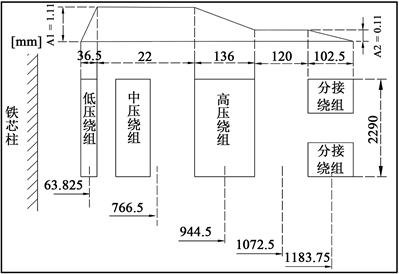Figure 6. Magnetic flux distribution schematic diagram for transformer H-L running in min. tapTable 6. Calculation of the area of magnetic flux leakage for transformer H-L running in min. tap

${{U}^{\prime }}_{kx}=\frac{24.8×0.98×433.03×636×0.915×2310.89}{229×362.9×{10}^{4}}%=17.03%$ (23)

4.6. 最大分接高–低短路阻抗计算

${A}_{2}={W}_{F}×{I}_{F}/F=64×393.65/275555.6=0.09$ (24)

${A}_{1}={W}_{1}×{I}_{F}/F=636×393.65/275555.6=0.91$ (25)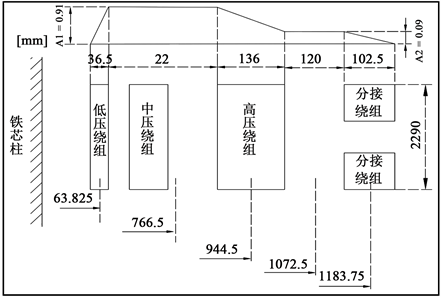Figure 7. Magnetic flux distribution schematic diagram for transformer H-L running in max. tapTable 7. Calculation of the area of magnetic flux leakage for transformer H-L running in max. tap

${{U}^{\prime }}_{kx}=\frac{24.8×0.98×433.03×636×0.915×2132.87}{229×362.9×{10}^{4}}%=15.72%$ (26)

4.7. 中–低短路阻抗计算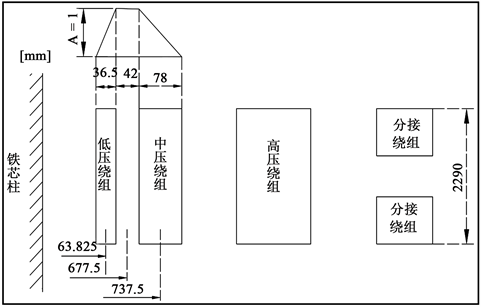Figure 8. Calculation of the area of magnetic flux leakage for transformer M-L runningTable 8. Calculation of the area of magnetic flux leakage for transformer M-L running

${{U}^{\prime }}_{kx}=\frac{24.8×0.98×433.03×636×0.978×709.26}{229×362.9×{10}^{4}}%=5.59%$ (27)

5. PPEN主变压器短路阻抗的匹配设计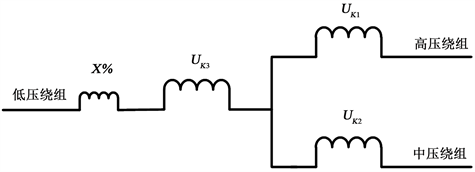Figure 9. Schematic diagram of series reactor in transformer low-voltage side

$X%={{U}^{\prime }}_{K3}-{U}_{K3}=\frac{\left({{U}^{\prime }}_{K23}+{{U}^{\prime }}_{K13}-{{U}^{\prime }}_{K12}\right)-\left({U}_{K23}+{U}_{K13}-{U}_{K12}\right)}{2}=8.43%$ (28)

$X=\frac{X%×{U}_{N3}}{{I}_{N3}}=\frac{8.43%×22500}{2222.2}=0.854\text{\hspace{0.17em}}\Omega$ (29)

$L=\frac{1000×X}{2\text{π}f}=\frac{1000×0.854}{2\text{π}×50}=2.72\text{\hspace{0.17em}}\text{mH}$ (30)

${P}_{L}=3×2\text{π}fL{I}_{N}^{2}=12.65\text{\hspace{0.17em}}\text{MVA}$ (31)

IEC 60076-1：2011表1中规定的短路阻抗的偏差：额定分接高–中为±10%，其他分接高–中为±15%，额定分接高–低为±7.5%，其他分接高–低为±10%，中–低为±10%  。所以，由表9数据可知，PPEN主变压器的短路阻抗分析计算结果、试验测量值以及它们的偏差均在IEC标准的偏差范围内，证明在低压绕组侧内置串联电抗器的短路阻抗匹配设计方法合理。Table 9. Calculation and test results for PPEN transformer short-circuit impedance

6. 结论

Design and Test Research on Short-Circuit Impedance for High Power Pulse Transformer[J]. 核科学与技术, 2019, 07(01): 16-27. https://doi.org/10.12677/NST.2019.71003

1. 1. 傅鹏. 国际聚变堆ITER装置电源系统综述[J]. 电力电子技术, 2014, 48(12): 1-7.

2. 2. 杨志刚, 傅鹏, 等. ITER脉冲功率变电站一次系统研究与实现[J]. 强激光与粒子束, 2017, 29(6): 1-6.

3. 3. 潘传红. 国际热核实验反应堆(ITER)计划与未来核聚变能源[J]. 物理, 2010, 39(6): 375-378.

4. 4. 尹克宁. 变压器设计原理[M]. 北京: 中国电力出版社, 2003.

5. 5. 路长柏. 电力变压器理论与计算[M]. 沈阳: 辽宁科学技术出版社, 2007.

6. 6. EI Hayek, J. (2001) Short-Circuit Reactances of Multi-Secondaries Concentric Windings Transformers. Electric Machines and Drives Conference, 462-465.

7. 7. 周雨. 一种220 kV高阻抗变压器工程设计新方法[D]: [硕士学位论文]. 保定: 华北电力大学, 2013.

8. 8. 杨志刚. ITER PPEN主变压器电磁设计及其特性分析[D]: [博士学位论文]. 合肥: 中国科学技术大学, 2017.

9. 9. 李洪春. 22 kV三绕组有载调压高阻抗变压器设计[J]. 变压器, 2009, 46(5): 1-11.

10. 10. IEC 60076-1: 2011, Power transformers—Part 1: General.